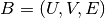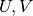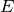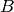NetworkX

#### Previous topic

attribute_mixing_dict

is_bipartite

# Bipartite¶

This module provides functions and operations for bipartite graphs. Bipartite graphshave two node setsand edges inthat only connect nodes from opposite sets. It is common in the literature to use an spatial analogy referring to the two node sets as top and bottom nodes.

The bipartite algorithms are not imported into the networkx namespace at the top level so the easiest way to use them is with:

```>>> import networkx as nx
>>> from networkx.algorithms import bipartite
```

NetworkX does not have a custom bipartite graph class but the Graph() or DiGraph() classes can be used to represent bipartite graphs. However, you have to keep track of which set each node belongs to, and make sure that there is no edge between nodes of the same set. The convention used in NetworkX is to use a node attribute named “bipartite” with values 0 or 1 to identify the sets each node belongs to.

For example:

```>>> B = nx.Graph()
>>> B.add_edges_from([(1,'a'), (1,'b'), (2,'b'), (2,'c'), (3,'c'), (4,'a')])
```

Many algorithms of the bipartite module of NetworkX require, as an argument, a container with all the nodes that belong to one set, in addition to the bipartite graph. Ifis connected, you can find the node sets using a two-coloring algorithm:

```>>> nx.is_connected(B)
True
>>> bottom_nodes, top_nodes = bipartite.sets(B)
>>> list(top_nodes)
[1, 2, 3, 4]
>>> list(bottom_nodes)
['a', 'c', 'b']
```

However, if the input graph is not connected, there are more than one possible colorations. Thus, the following result is correct:

```>>> B.remove_edge(2,'c')
>>> nx.is_connected(B)
False
>>> bottom_nodes, top_nodes = bipartite.sets(B)
>>> list(top_nodes)
[1, 2, 4, 'c']
>>> list(bottom_nodes)
['a', 3, 'b']
```

Using the “bipartite” node attribute, you can easily get the two node sets:

```>>> top_nodes = set(n for n,d in B.nodes(data=True) if d['bipartite']==0)
>>> bottom_nodes = set(B) - top_nodes
>>> list(top_nodes)
[1, 2, 3, 4]
>>> list(bottom_nodes)
['a', 'c', 'b']
```

So you can easily use the bipartite algorithms that require, as an argument, a container with all nodes that belong to one node set:

```>>> print(round(bipartite.density(B, bottom_nodes),2))
0.42
>>> G = bipartite.projected_graph(B, top_nodes)
>>> G.edges()
[(1, 2), (1, 4)]
```

All bipartite graph generators in NetworkX build bipartite graphs with the “bipartite” node attribute. Thus, you can use the same approach:

```>>> RB = nx.bipartite_random_graph(5, 7, 0.2)
>>> nx.is_connected(RB)
False
>>> RB_top = set(n for n,d in RB.nodes(data=True) if d['bipartite']==0)
>>> RB_bottom = set(RB) - RB_top
>>> list(RB_top)
[0, 1, 2, 3, 4]
>>> list(RB_bottom)
[5, 6, 7, 8, 9, 10, 11]
```

For other bipartite graph generators see the bipartite section of Graph generators.

## Basic functions¶

 is_bipartite(G) Returns True if graph G is bipartite, False if not. is_bipartite_node_set(G, nodes) Returns True if nodes and G/nodes are a bipartition of G. sets(G) Returns bipartite node sets of graph G. color(G) Returns a two-coloring of the graph. density(B, nodes) Return density of bipartite graph B. degrees(B, nodes[, weight]) Return the degrees of the two node sets in the bipartite graph B. biadjacency_matrix(G, row_order[, ...]) Return the biadjacency matrix of the bipartite graph G.

## Projections¶

One-mode (unipartite) projections of bipartite graphs.

 projected_graph(B, nodes[, multigraph]) Returns the projection of B onto one of its node sets. weighted_projected_graph(B, nodes[, ratio]) Returns a weighted projection of B onto one of its node sets. collaboration_weighted_projected_graph(B, nodes) Newman’s weighted projection of B onto one of its node sets. overlap_weighted_projected_graph(B, nodes[, ...]) Overlap weighted projection of B onto one of its node sets. generic_weighted_projected_graph(B, nodes[, ...]) Weighted projection of B with a user-specified weight function.

## Spectral¶

Spectral bipartivity measure.

 spectral_bipartivity(G[, nodes, weight]) Returns the spectral bipartivity.

## Clustering¶

 clustering(G[, nodes, mode]) Compute a bipartite clustering coefficient for nodes. average_clustering(G[, nodes, mode]) Compute the average bipartite clustering coefficient.

## Redundancy¶

Node redundancy for bipartite graphs.

 node_redundancy(G[, nodes]) Compute bipartite node redundancy coefficient.

## Centrality¶

 closeness_centrality(G, nodes[, normalized]) Compute the closeness centrality for nodes in a bipartite network. degree_centrality(G, nodes) Compute the degree centrality for nodes in a bipartite network. betweenness_centrality(G, nodes) Compute betweenness centrality for nodes in a bipartite network.